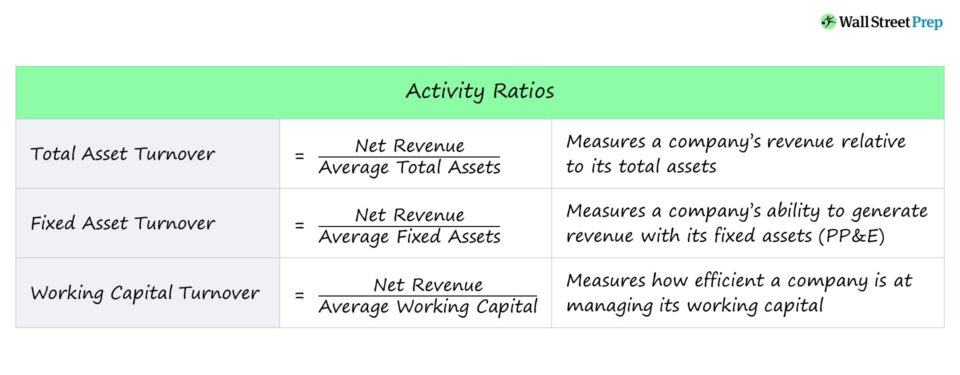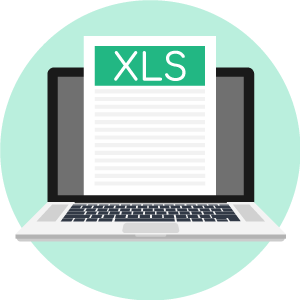# Activity Ratio

Guide to Understanding the Activity Ratio Concept• What is an activity ratio?
• What are some examples of common activity ratios?
• What are activity ratios used to measure?
• What are the formulas to calculate the

## How to Calculate an Activity Ratio

The efficiency at which a company utilizes its assets can be measured by activity ratios.

An activity ratio is an indicator of how efficient a company is at asset allocation, with the goal of deriving as much revenue as possible with the least amount of resources.

One can gauge a company’s ability to manage its current assets such as inventory and accounts receivable as well as fixed assets (PP&E) to generate more revenue.

Therefore, by comparing the two sides — revenue and an asset metric — each “turnover” ratio measures the relationship between the two and how they trend over time.

## Activity Ratio Formula

Each activity ratio consists of revenue in the numerator and then a measure of an asset(s) in the denominator.

###### Activity Ratio Formulas
• Total Asset Turnover Ratio = Revenue / Average Total Assets
• Fixed Asset Turnover Ratio = Revenue / Average Fixed Assets
• Working Capital Turnover Ratio = Revenue / Average Working Capital

## Inventory, Receivables and Payables Turnover Ratio

As a general rule of thumb, the higher the turnover ratio, the better — since it implies the company can generate more revenue with fewer assets.

The majority of companies closely track their accounts receivable (A/R) and inventory trends; hence, these accounts are frequently used in the denominator of activity ratios.

While there are numerous variations of activity ratios such as the accounts receivable turnover ratio and inventory turnover ratio, the shared purpose of each ratio is to determine how well a company can utilize its operating assets.

An improvement in activity ratios tends to correspond with higher profit margins, since more value is extracted from each asset.

Some of the more common ratios are:

• Inventory Turnover — The number of times a company’s inventory is replenished in a specific period
• Receivables Turnover Ratio — The number of times a typical customer that originally paid on credit (i.e. accounts receivable, or “A/R”) makes a cash payment in a specific period
• Payables Turnover Ratio — The number of times a company pays off its due payments to suppliers/vendors (i.e. accounts payable, or “A/P”) in a specific period

### Other Activity Ratio Formulas

• Inventory Turnover = Cost of Goods Sold (COGS) / Average Inventory
• Receivables Turnover = Revenue / Average Accounts Receivable (A/R)
• Payables Turnover Ratio = Total Credit Purchases / Average Accounts Payable

## Activity Ratios vs Profitability Ratios

Both activity ratios and profitability ratios should be analyzed to determine a company’s financial health.

Profitability ratios such as the gross margin and operating margin help depict a company’s overall ability to convert revenue into earnings after accounting for different costs/expenses.

In comparison, activity ratios measure a company’s ability to efficiently use its resources (i.e. assets) to generate profits, just on a more granular level (i.e. per asset).

## Activity Ratio Calculator – Excel Template

We’ll now move to a modeling exercise, which you can access by filling out the form below.Submitting ...

## Activity Ratios Example Calculation

Here in our illustrative example, we’ll be projecting three activity ratios — the total asset turnover, fixed asset turnover, and working capital turnover ratios — across five years.

As of Year 0, the financial assumptions to be used are shown below, with the year-over-year (YoY) growth assumptions to the right.

###### Model Assumptions — Financial Data
• Revenue = \$100m with +\$20m Increase Per Year
• Cash & Equivalents = \$25m with +\$5m Increase Per Year
• Accounts Receivable = \$45m with -\$2m Decrease Per Year
• Inventory = \$60m with -\$2m Decrease Per Year
• Property, Plant & Equipment (PP&E) = \$225m with -\$5m Decrease Per Year
• Accounts Payable (A/P) = \$50m with +\$5m Increase Per Year
• Accrued Expenses = \$10m with +\$1m Increase Per Year

Using the assumptions provided, we can first calculate the total asset turnover ratio in Year 1 by dividing the current revenue by the average between the current and prior period total asset balance.In the subsequent steps, we can repeat the process for the fixed asset turnover and the working capital turnover — with the denominator as the only changing variable.

Starting from Year 0 to the end of the forecast period in Year 5, the following changes occur:

1. Total Asset Turnover Ratio: 0.3x → 0.6x
2. Fixed Asset Turnover Ratio: 0.5x → 1.0x
3. Working Capital Turnover Ratio: 1.8x → 4.2x

Interpreting the changes is predicated on the industry that our company operates in, as well as other company-specific factors that are beyond the scope of our simple modeling exercise.

However, based on the limited information available, our company’s “top line” revenue is growing by \$20m each year while its cash balance is increasing by \$5m.

Furthermore, A/R and inventory — metrics that measure the amount of cash tied up in operations — are declining each year, which implies the company is collecting cash payments from customers that paid on credit and clearing out inventory faster.

On the other side of the balance sheet, the increasing accounts payable balance can be perceived as a positive trend signifying increased negotiating leverage over suppliers (i.e. suppliers allowing for days payable outstanding to extend).Step-by-Step Online Course

#### Everything You Need To Master Financial Modeling

Enroll in The Premium Package: Learn Financial Statement Modeling, DCF, M&A, LBO and Comps. The same training program used at top investment banks.Inline FeedbacksLearn Financial Modeling Online

Everything you need to master financial and valuation modeling: 3-Statement Modeling, DCF, Comps, M&A and LBO.

X

The Wall Street Prep Quicklesson Series

7 Free Financial Modeling Lessons

Get instant access to video lessons taught by experienced investment bankers. Learn financial statement modeling, DCF, M&A, LBO, Comps and Excel shortcuts.